# Volume 28, 2022, Number 1

Volume 28 ▶ Number 1 ▷ Number 2Number 3Number 4

Character formulas in terms of Rβ and Rm functions
Original research paper. Pages 1—8
M. P. Chaudhary, Sangeeta Chaudhary and Kamel Mazhouda

The authors establish a set of fourteen character formulas in terms of Rβ and Rm functions. Folsom  studied character formulas and Chaudhary  expressed those formulas in terms of continued fraction identities. Andrews et al.  introduced multivariate R-functions, which are further classified as Rα; Rβ, and Rm (for m = 1, 2, 3, …) functions by Srivastava et al. .

On the Vieta–Jacobsthal-like polynomials
Original research paper. Pages 9—19
Wanna Sriprad, Somnuk Srisawat and Kitsana Charoenchaianan

In this paper, we first introduce the generalization of the Vieta–Jacobsthal polynomial, which is called the Vieta–Jacobsthal-like polynomial. After that, we give the generating function, the Binet formula, and some well-known identities for this polynomial. Finally, we also present the relation between this polynomial and the previously famous Vieta polynomials.

Corrigendum to “On the dimension of an Abelian group” [Notes on Number Theory and Discrete Mathematics, 2022, Volume 27, Number 4, Pages 267—275]
Corrigendum. Page 20
Timo Tossavainen and Pentti Haukkanen

Factorials as repdigits in base b
Original research paper. Pages 21—25
Nurettin Irmak and Alain Togbé

Let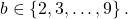In this paper, we show that the solutions of the equation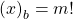are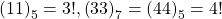, where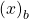has at least two digits.

Multicomponent hybrid numbers: On algebraic properties and matrix representations of hybrid-hyperbolic numbers
Original research paper. Pages 26—40
Bahar Doğan Yazıcı and Murat Tosun

In this study, the hybrid-hyperbolic numbers are introduced. This number system is a more general form of the hybrid number system, which is an interesting number system, as well as a number system that includes multicomponent number systems (i.e., complex-hyperbolic, dual-hyperbolic and bihyperbolic numbers). In this paper, we give algebraic properties of hybrid-hyperbolic numbers. In addition, 2 × 2 and 4 × 4 hyperbolic matrix representations of hybrid-hyperbolic numbers are given and some properties of them are examined.

The amplitude of Motzkin paths
Original research paper. Pages 41—47
Helmut Prodinger

The amplitude of Motzkin paths was recently introduced, which is basically twice the height. We analyze this parameter using generating functions.

Cycles of higher-order Collatz sequences
Original research paper. Pages 48—63
John L. Simons

Consider a sequence of numbers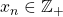defined by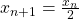if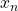is even, and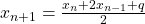ifis odd. A-cycle is a periodic sequence with one transition from odd to even numbers. We prove theoretical and computational results for the existence of-cycles, and discuss a generalization to more complex cycles.

Determinantal and permanental representations of companion sequences associated to the r-Fibonacci sequence
Original research paper. Pages 64—74
Hacène Belbachir and Ihab-Eddine Djellas

Recently companion sequences of r-Fibonacci sequence were defined. The aim of this paper is to give some determinantal and permanental representations of these sequences via Hessenberg matrices. Several representations of classical sequences and polynomials are established. We conclude by using our representations to give n consecutive terms of companion sequences simultaneously.

Two theorems on square numbers
Original research paper. Pages 75—80
Nguyen Xuan Tho

We show that if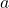is a positive integer such that for each positive integer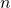,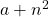can be expressed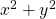, where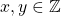, thenis a square number. A similar theorem also holds ifandare replaced by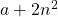and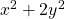, respectively.

Set partitions with isolated successions
Original research paper. Pages 81—91
Toufik Mansour and Augustine O. Munagi

We enumerate partitions of the set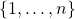according to occurrences of isolated successions, that is, integer strings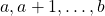in a block when neither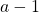nor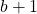lies in the same block. Our results include explicit formulas and generating functions for the number of partitions containing isolated successions of a given length.
We also consider a corresponding analog of the associated Stirling numbers of the second kind.

Sums involving generalized harmonic and Daehee numbers
Original research paper. Pages 92—99
Neşe Ömür and Sibel Koparal

In this paper, we establish some sums involving generalized harmonic and Daehee numbers which are derived from the generating functions. For example, for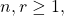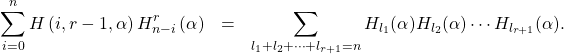Partitions with k sizes from a set
Original research paper. Pages 100—108
A. David Christopher

Letand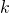be two positive integers and letbe a set of positive integers. We define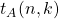to be the number of partitions ofwith exactlysizes and parts in. As an implication of a variant of Newton’s product-sum identities we present a generating function for. Subsequently, we obtain a recurrence relation forand a divisor-sum expression for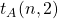. Also, we present a bijective proof for the latter expression.

A note on generalized and extended Leonardo sequences
Original research paper. Pages 109—114
Anthony G. Shannon and Ömür Deveci

This note considers some real and complex extensions and generalizations of the Leonardo sequence, which is embedded within each of these two types of intriguing sequences, intriguing because there are still some unanswered questions. The connections between inhomogeneous and homogeneous forms are used as examples of a possible reason that the Leonardo sequences have been, in a sense, historically neglected.

Leonardo’s bivariate and complex polynomials
Original research paper. Pages 115—123
Milena Carolina dos Santos Mangueira, Renata Passos Machado Vieira, Francisco Regis Vieira Alves and Paula Maria Machado Cruz Catarino

Given the purpose of mathematical evolution of Leonardo’s sequence, we have the prospect of introducing complex polynomials, bivariate polynomials and bivariate polynomials around these numbers. Thus, this article portrays in detail the insertion of the variable x, y and the imaginary unit i in the sequence of Leonardo. Nevertheless, the mathematical results from this process of complexification of these numbers are studied, correlating the mathematical evolution of that sequence.

On certain inequalities for the prime counting function – Part II
Original research paper. Pages 124—128
József Sándor

As a continuation of , we deduce some inequalities of a new type for the prime counting function π(x).

Linear recurrence sequence associated to rays of negatively extended Pascal triangle
Original research paper. Pages 129—142
Hacène Belbachir, Abdelkader Bouyakoub and Fariza Krim

We consider the extension of generalized arithmetic triangle to negative values of rows and we describe the recurrence relation associated to the sum of diagonal elements laying along finite rays. We also give the corresponding generating function. We conclude by an application to Fibonacci numbers and Morgan-Voyce polynomials with negative subscripts.

On two new combined 3-Fibonacci sequences. Part 3
Original research paper. Pages 143—146
Krassimir T. Atanassov